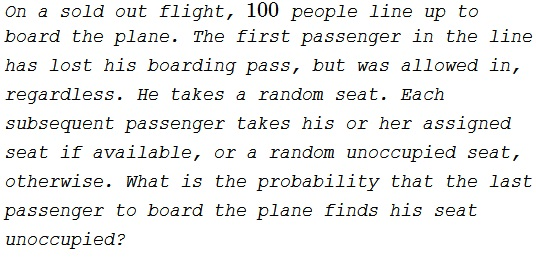# The Lost Boarding Pass

### Problem### Solution 1

When the last passenger boards the plane, there are just two possibilities: the one remaining seat may be his or that of the first passenger. Under the assumption that no preference has been exhibited by the boarding passengers towards either of the two seats, they both have the same probability to become the last unoccupied seat: 50%.

### Solution 2

Suppose there are $n \ge 2$ passengers and $n$ seats. If, for any $k \lt n,$ the first $k$ passengers occupy assigned to them (as a group, not individually) then from then on everybody, including the last passenger, sits on his own seat. Also, if one of the first $k \lt n$ passengers occupies the seat of the last passenger then the last passenger will not sit in his own seat. Now, if, for $k - 1,$ neither of these events holds then the $k^{th}$ passenger's choice has exactly as much chance of leading to the first event as to the second: if his own seat is unoccupied, neither of the events will happen, and if his own seat seat is occupied then there is exactly one (unoccupied) seat, the first, that leads to the first event and exactly one (unoccupied) seat, the last, that leads to the second.

B. Bollobás notes that this question was on Car Talk, the popular NPR program airing on Saturdays, and was brought to his attention by Oliver Riordan, who also gave the proof above. Also, note that the full plane is important. If there are more seats than the passengers then it is more likely that the last passenger sits in his own seat.

### Solution 3

There is $\displaystyle \frac{1}{100}$ chance the first passenger sits on his own sit, then the last passenger's sit will be available for sure; $\displaystyle \frac{1}{100}$ chance to sit on the last passenger's sit - then it will be occupied for sure; and $\displaystyle \frac{98}{100}$ chance to sit on someone's other sit and, whenever that happens, the first "middle" passenger who takes either first or last passenger's sit will do that with $\displaystyle \frac{1}{2}$ probability for each. So:

$\displaystyle \frac{1}{100} \cdot 1 + \frac{98}{100} \cdot \frac{1}{2} + \frac{1}{100} \cdot 0 = \frac{1}{2}.$

### Solution 4

Define:

$A_k$: Event that kth passenger sits in his own seat.
$I_m$: Event that 1st passenger took mth seat.

Then,

\displaystyle\begin{align} P(A_k)&=\frac{n-k+1}{n}\cdot 1+\frac{1}{n}\cdot0+\frac{k-2}{n}\cdot\frac{n-k+1}{n-k+2}\\ &=\frac{n-k+1}{n(n-k+2)}[(n-k+2)+(k-2)]\\ &=\frac{n-k+1}{n-k+2}. \end{align}

E.g., for $n=8,\,k=6,\,m=1\ldots,8,$

• $I_1, I_6, I_7, I_8$ events are obvious.

• $I_5$ event: Then $P(A_2)=P(A_3)=P(A_4)=1$, so 5th person has only 4 seats available, $P(A_6|I_5)=3/4$

• $I_4$ event: Then $P(A_2)=P(A_3)=1$, so we have 2 cases:

1st $I_4$ case: 4th person goes to seat #5, then 5th person has only 4 seats available, $P(A_6|I_4)=3/4$

2nd $I_4$ case: 4th person goes to a seat different than #5, then $P(A_5)=1$, 4 seats available, $P(A_6|I_4)=3/4$

• $I_3$ event: Then $P(A_2)=1$, so we have 3 cases:

1st $I_3$ case: 3rd person goes to seat #4, then we proceed similarly with $I_4$ event, $P(A_6|I_3)=3/4$

2nd $I_3$ case: 3rd person goes to seat #5, then $P(A_4)=1$, then we proceed similarly with $I_5$ event, $P(A_6|I_3)=3/4$

3rd $I_3$ case: 3rd person goes to a seat different than #4 or #5, then $P(A_4)=P(A_5)=1$, 4 seats available, $P(A_6|I_3)=3/4$

• $I_2$ event: With similar reasoning as above we have 4 cases and $P(A_6|I_2)=3/4$

$\displaystyle P(A_6)=\sum_{m=1}^8P(A_6|I_m)=\frac{1}{8}\cdot\left(1+\frac{3}{4}+\frac{3}{4}+\frac{3}{4}+\frac{3}{4}+0+1+1\right)=\frac{3}{4}$

and

$\displaystyle P(A_6^c)=1-\frac{3}{4}=\frac{1}{4}$

Similarly for $k=8,$

$\displaystyle P(A_8)=\frac{1}{8}\cdot 1+\frac{1}{8}\cdot0+\frac{6}{8}\cdot\frac{1}{2}=\frac{1}{2}$

### Acknowledgment

Solution 1 is from P. Winkler's Mathematical Puzzles: A Connoisseur's Collection (A K Peters, 2004, pp. 35-37).

Solution 2 is from B. Bollobás' The Art of Mathematics: Coffee Time in Memphis (Cambridge University Press, 2006, p. 176.)

Solution 3 is by Sergej Saletic; Solution 4 is by Giorgos Papadopulos.

There is a related page with the question of the average number of misplaced passengers.

Jim Totten (in J. G. McLoughlin et all, Jim Totten's Problems of the Week, World Scientific, 2013, problem #324) considers the situation when the passengers board the plane in numerical order. The final answer is the same $\displaystyle \frac{1}{2}$ proved by induction.